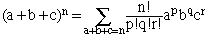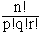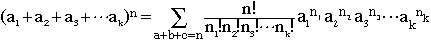Hi my name is murray and I am a highschool student. Could you please state and explain the multinomial theorem (I already know the binomial theorem etc, to give you an idea of where i am) Thanks alot Hi Murray, Since you know the binomial theorem I would start with trinomials. You should expand (a + b + c)2 and (a + b + c)3 by multiplying them out to see how the coefficients are constructed. (a + b + c)2 =(a + b + c)(a + b + c) = a2 + b2 + c2 + 2ab + 2ac + 2bc (a + b + c)3 = (a + b + c)(a + b + c)(a + b + c) = a3 + b3 + c3 + 3a2b + 3a2c + 3ab2 + 3b2c + 3 ac2 + 3 bc2 + 6 abc If you think of these two examples as (a + b + c)n then each of the term is a constant times apbqcr where p + q + r = n. In the first case n = 2 and in the second n = 3. In the general caseThe notation means that you form a sum of all possible terms of the form apbqcr where p + q + r = n with each term having a coefficient ofThe multinomial theorem is of the same form.Cheers, Harley Go to Math Central